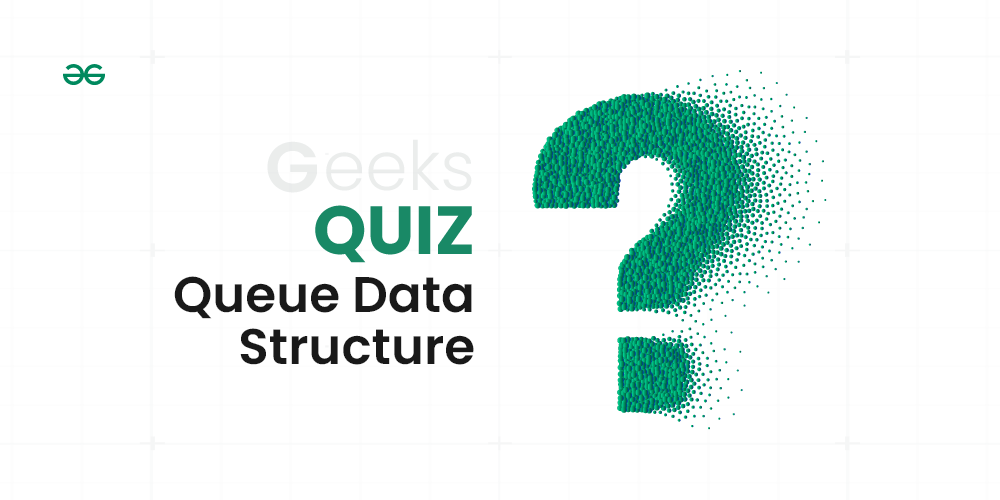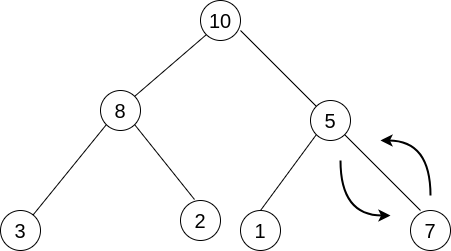# Top MCQs on Queue Data Structure with Answers

A Queue is defined as a linear data structure that is open at both ends and the operations are performed in First In First Out (FIFO) order.
More On Queue Data StructureQueue Data Structure Quiz

 Question 1

Following is C like pseudo-code of a function that takes a Queue as an argument, and uses a stack S to do processing.

## C

```void fun(Queue *Q)
{
Stack S;  // Say it creates an empty stack S

// Run while Q is not empty
while (!isEmpty(Q))
{
// deQueue an item from Q and push the dequeued item to S
push(&S, deQueue(Q));
}

// Run while Stack S is not empty
while (!isEmpty(&S))
{
// Pop an item from S and enqueue the popped item to Q
enQueue(Q, pop(&S));
}
}
```

What does the above function do in general?Removes the last from QKeeps the Q same as it was before the callMakes Q emptyReverses the Q

Question 1-Explanation:

The function takes a queue Q as an argument. It dequeues all items of Q and pushes them to a stack S. Then pops all items of S and enqueues the items back to Q. Since the stack is LIFO order, all items of the queue are reversed.

Hence option (D) is the correct answer.

 Question 2

Which one of the following is an application of Queue Data Structure?When a resource is shared among multiple consumers.When data is transferred asynchronously (data not necessarily received at same rate as sent) between two processesLoad BalancingAll of the above

Question 2-Explanation:

(A) When a resource is shared among multiple consumers: In scenarios where a resource (such as a printer, CPU time, or database connection) needs to be shared among multiple consumers or processes, a queue data structure can be used. Each consumer can enqueue their requests for the resource, and the resource can be allocated to them in the order of their requests by dequeuing from the queue. This ensures fair access to the shared resource and prevents conflicts or resource contention.

(B) When data is transferred asynchronously between two processes: When data is transferred asynchronously between two processes or systems, a queue can be used as a buffer or intermediary storage. One process enqueues the data to be sent, while the other process dequeues and processes the received data. The queue allows for decoupling the rate of data production from data consumption, ensuring smooth and efficient communication between the processes.

(C) Load Balancing: Load balancing is the practice of distributing workloads across multiple resources to optimize performance and utilization. A queue data structure can be used in load-balancing algorithms to manage incoming requests or tasks. The requests are enqueued in the queue, and the load balancer can dequeue and assign them to available resources based on various criteria (e.g., round-robin, least connections). This helps distribute the workload evenly across the resources, preventing overload and maximizing throughput.

Hence (D) is the correct option.

 Question 3

How many stacks are needed to implement a queue. Consider the situation where no other data structure like arrays, linked list is available to you.1234

Question 3-Explanation:

A queue can be implemented using two stacks.

Refer this for more reference:
https://www.geeksforgeeks.org/queue-using-stacks/

Hence Option(B) is the correct answer.

 Question 4

How many queues are needed to implement a stack. Consider the situation where no other data structure like arrays, linked list is available to you.1234

Question 4-Explanation:

A stack can be implemented using two queues.

Refer this for more reference: https://www.geeksforgeeks.org/implement-stack-using-queue/

Hence Option(B) is the correct answer.

 Question 5

A priority queue can efficiently implemented using which of the following data structures? Assume that the number of insert and peek (operation to see the current highest priority item) and extraction (remove the highest priority item) operations are almost same.ArrayLinked ListHeap Data Structures like Binary Heap, Fibonacci HeapNone of the above

Question 5-Explanation:

A priority queue can be efficiently implemented using a data structure called a binary heap or Fibonacci Heap. A binary heap is a complete binary tree that satisfies the heap property. Binary heaps provide excellent time complexity for these operations, making them an efficient choice for implementing priority queues when the number of insert and peek/extraction operations is roughly equal.

Hence option(C) is the correct answer.

 Question 6

Which of the following is true about linked list implementation of queue?In push operation, if new nodes are inserted at the beginning of linked list, then in pop operation, nodes must be removed from end.In push operation, if new nodes are inserted at the end, then in pop operation, nodes must be removed from the beginning.Both of the aboveNone of the above

Question 6-Explanation:

To keep the First In First Out order, a queue can be implemented using a linked list in any of the given two ways.

Hence option (C) is the correct answer.

 Question 7

Suppose a circular queue of capacity (n – 1) elements is implemented with an array of n elements. Assume that the insertion and deletion operation are carried out using REAR and FRONT as array index variables, respectively. Initially, REAR = FRONT = 0. The conditions to detect queue full and queue empty areFull: (REAR+1) mod n == FRONT, empty: REAR == FRONTFull: (REAR+1) mod n == FRONT, empty: (FRONT+1) mod n == REARFull: REAR == FRONT, empty: (REAR+1) mod n == FRONTFull: (FRONT+1) mod n == REAR, empty: REAR == FRONT

Question 7-Explanation:

Suppose we start filling the queue.

Let the maxQueueSize ( Capacity of the Queue) is 4.So the size of the array which is used to implement this circular queue is 5, which is n. In the beginning when the queue is empty, FRONT and REAR point to 0 index in the array. REAR represents insertion at the REAR index. FRONT represents deletion from the FRONT index.

enqueue("a"); REAR = (REAR+1)%5; ( FRONT = 0, REAR = 1)

enqueue("b"); REAR = (REAR+1)%5; ( FRONT = 0, REAR = 2)

enqueue("c"); REAR = (REAR+1)%5; ( FRONT = 0, REAR = 3)

enqueue("d"); REAR = (REAR+1)%5; ( FRONT = 0, REAR = 4)

Now the queue size is 4 which is equal to the maxQueueSize. Hence overflow condition is reached.

Now, we can check for the conditions.

When Queue Full :
( REAR+1)%n = (4+1)%5 = 0
FRONT is also 0. Hence ( REAR + 1 ) %n is equal to FRONT.

When Queue Empty :

REAR was equal to FRONT when empty ( because in the starting before filling the queue FRONT = REAR = 0 )

Hence Option A is correct.

 Question 8

A Priority-Queue is implemented as a Max-Heap. Initially, it has 5 elements. The level-order traversal of the heap is given below: 10, 8, 5, 3, 2 Two new elements ”1‘ and ”7‘ are inserted in the heap in that order. The level-order traversal of the heap after the insertion of the elements is:10, 8, 7, 5, 3, 2, 110, 8, 7, 2, 3, 1, 510, 8, 7, 1, 2, 3, 510, 8, 7, 3, 2, 1, 5

Question 8-Explanation:

The output will be 10, 8,  7, 3, 2, 1, 5.

Refer the image for clarification:Hence Option(D) is the correct answer.

 Question 9

An implementation of a queue Q, using two stacks S1 and S2, is given below:

## C

```void insert(Q, x) {
push (S1, x);
}

void delete(Q){
if(stack-empty(S2)) then
if(stack-empty(S1)) then {
print(“Q is empty”);
return;
}
else while (!(stack-empty(S1))){
x=pop(S1);
push(S2,x);
}
x=pop(S2);
}
```

Let n insert and m (<=n) delete operations be performed in an arbitrary order on an empty queue Q. Let x and y be the number of push and pop operations performed respectively in the process. Which one of the following is true for all m and n?n+m <= x < 2n and 2m <= y <= n+mn+m <= x < 2n and 2m<= y <= 2n2m <= x < 2n and 2m <= y <= n+m2m <= x <2n and 2m <= y <= 2n

Question 9-Explanation:

The order in which insert and delete operations are performed matters here. The best case: Insert and delete operations are performed alternatively. In every delete operation, 2 pop and 1 push operations are performed. So, total m+ n push (n push for insert() and m push for delete()) operations and 2m pop operations are performed. The worst case: First n elements are inserted and then m elements are deleted. In first delete operation, n + 1 pop operations and n push operation are performed. Other than first, in all delete operations, 1 pop operation is performed. So, total m + n pop operations and 2n push operations are performed (n push for insert() and n push for delete())

Hence Option (A) is the correct answer.

 Question 10

Consider the following operation along with Enqueue and Dequeue operations on queues, where k is a global parameter.

```MultiDequeue(Q){
m = k
while (Q is not empty and m  > 0) {
Dequeue(Q)
m = m - 1
}
}```

What is the worst case time complexity of a sequence of n MultiDequeue() operations on an initially empty queue? (GATE CS 2013) (A)(B)(C)(D)ABCD

Question 10-Explanation:

Since the queue is empty initially, the condition of while loop never becomes true. So the time complexity is.

Hence Option (A) is the correct answer.

There are 30 questions to complete.

• Last Updated : 27 Sep, 2023